Courses

NCERT Solutions - Gravitation - Science Class 9 Notes - Class 9

Class 9: NCERT Solutions - Gravitation - Science Class 9 Notes - Class 9

The document NCERT Solutions - Gravitation - Science Class 9 Notes - Class 9 is a part of Class 9 category.
All you need of Class 9 at this link: Class 9
Chapter 10, GRAVITATION

Q.1: How does the force of gravitation between two objects change when the distance between them is reduced to half?

Ans: According to the law of gravitation, the force of attraction acting between two bodies is given by,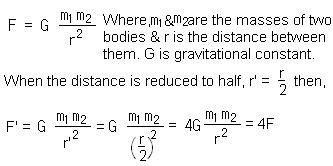Thus, when the distance between the objects is reduced to half the gravitational force increases by four times the original force.

Q.2: The gravitational force acts on all objects in proportion to their masses. Why, then, a heavy object does not fall faster than a light object?
Ans: Let F be the gravitational force acting on a body of mass m, then it can be given by,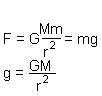From the above equations we can see that F α m, although g (acceleration due to gravity) does not depend on m. Hence, all bodies fall with the same acceleration provided there is no air or other resistance.

Q.3: What is the magnitude of the gravitational force between the earth and a 1 kg object on its surface? (Mass of the earth = 6 x 1024 kg and radius of the earth is 6.4 x 106 m)
Ans: Given that,
Mass of the body (m) = 1 kg
Mass of the Earth (M) = 6 x 1024 kg
Radius of the earth (R) = 6.4 x 106 m
Now magnitude of the gravitational force (F) between the earth and the body can be given as,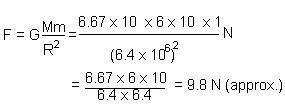Q.4: The earth and the moon are attracted to each other by gravitational force. Does the earth attract the moon with a force that is greater or smaller or the same as the force with which the moon attracts the earth? Why?
Ans: The earth surface attracts the moon with the same force with which the moon attracts the earth. According to Newton’s 3rd law, these two forces are equal and opposite.
Also by the universal law of gravitation, the force acting between earth and moon will be: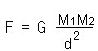Where M1 = Mass (earth), M2 = Mass (moon), d = Distance between earth and moon. The magnitude of force (F) is same for earth and moon.

Q.5: If the moon attracts the earth, why does the earth not move towards the moon?

Ans: According to the Newton’s 3rd law of motion, the moon also attracts the earth with a force equal to that with which the earth attracts the moon. Since the earth is much larger than the moon so, the acceleration produced on the earth surface (acceleration α 1/m) can not be noticed.

Q.6: What happens to the force between two objects, if

(i) the mass of one object is doubled?
(ii) the distance between the objects is doubled and tripled?
(iii) the masses of both objects are doubled?
Ans:
(i) When the mass of either body is doubled, the force is also doubled as per the following equation: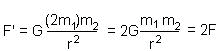(ii) When the distance between the objects is doubled, the force becomes one fourth of the original force as per the following equation: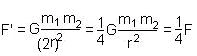Similarly it can be shown that when the distance between the objects is tripled, the force becomes one ninth of the original force.
(iii) In the same way as given in the answer (i) above this can be shown that when the masses of both bodies are doubled, the force becomes four times the original force.

Q.7: What is the importance of Universal Law of Gravitation?
Ans: There are many importance of Universal Law of Gravitation as with the help of this law we can explain natural phenomena such as,
1. the force of attraction that binds us to the earth,
2. the motion of planets moving around the sun,
3. the motion of moon around the earth
4. the occurring of tides due to sun and moon.

Q.8: What is the acceleration of free fall?
Ans: It is the acceleration produced when a body falls under the influence of the force of gravitation of the earth alone. On the surface of the earth its value is 9.8 m/s2.

Q.9: What do we call the gravitational force between the earth and an object?

Ans: Weight of that object.

Q.10: Amit buys few grams of gold at poles as per the instruction of one of his friends. He hands over the same when he meets him at equator. Will the friend agree with the weight of gold bought? If not, why?
Ans: His friend won’t agree with the weight of gold bought. Since the value of is less at the equator than that at the poles so, the same gold weighed at the poles will measure less when taken to equator.

Q.11: Why will a sheet of paper fall slower than one that is crumpled into a ball?
Ans: Because the sheet of paper will experience larger air resistance due to its larger surface area than its surface area in ball form. Hence, a sheet of paper falls slower than one that is crumpled into a ball.

.13: A ball is thrown vertically upwards with a velocity of 49 m/s. Calculate
(i) the maximum height to which it rises,
(ii) the total time it takes to return to the surface of the earth.
Ans: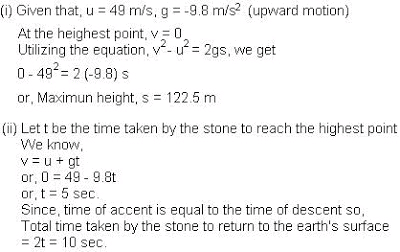Q.14: A stone is released from the top of a tower of height 19.6 m. calculate its final velocity just before touching the ground.
Ans: Given that,
u = 0, g = 9.8 ms–2, s = 19.6 m
Now, v2 - u2 = 2gs
or,     v2 - 0 = 2 x 9.8 x 19.6 = (19.6)2
or,     v = 19.6 ms–1 (we will consider value of v only +ve since it is in the downward direction)

Q.15: A stone is thrown vertically upwards with an initial velocity of 40 m/s. Taking g = 10 m/s2, find the maximum height reached by the stone. What is the net displacement and total distance covered by the stone?
Ans: Given that, u = 40 ms–1, g = –10 ms–2
At the highest point (h) the velocity (v) will be zero.
using the equation, v2 - u2 = 2gh
0 – 402 = 2(–10)h
or, h = 80 m
or, the total distance covered  = 2h = 160 m
Net displacement = 80 – 80 = 0

Q.16: Calculate the force of gravitation between the earth and the sun, given that the mass of the earth = 6 x 1024 kg and of the sun = 2 x 1030kg. The average distance between the two is 1.5 x 1011 m.

Ans: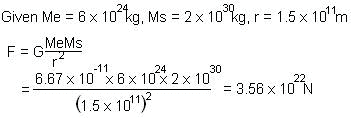Q.17: A stone is allowed to fall from the top of a tower 100 m high and at the same time another stone is projected vertically upwards from the ground with a velocity of 25 m/s. Calculate when and where the two stones will meet?
Ans: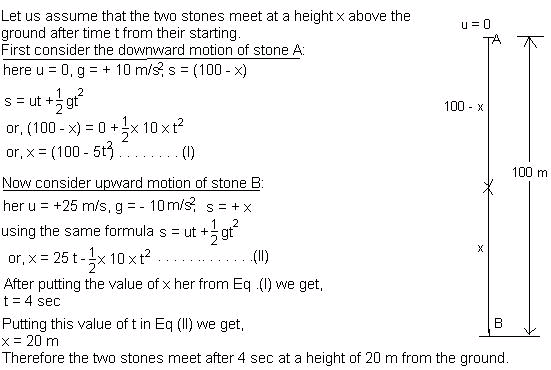Q.19: In what direction does the buoyant force on an object immersed in a liquid act?
Ans: The buoyant force acts on an object in the vertically upward direction through the center of gravity of the displaced liquid.

Q.20: Why does a block of plastic released under water come up to the surface of water?

Ans: It is because the upthrust or the buoyant force exerted by the water brings the plastic block to the surface of water.

Q.21: The volume of 50 gm of a substance is 20 cm3. If the density of water is 1 g/cm3, will the substance float or sink?

Ans:

Given the mass of the substance = 50 g
Volume of the substance = 20 cm3
Density of substance = Mass ÷ Volume = (50 ÷ 20) g/cm3 = 2.5 g/cm3
Since the density of the substance is greater than the density of water (1 g/cm3), so the substance will sink in the water.

Q.22: The volume of a 50 gm sealed packet is 350 cm3. Will the packet float or sink in water if the density of water is 1 g/cm3 ? What will be the mass of the water displaced by this packet ?

Ans:
Mass of packet = 500 g
Volume of packet = 350 cm3
Density of packet = Mass ÷ Volume = (500 ÷ 350) g/cm3 = 1.43 g/cm3
The sealed packet will sink in the water as its density is more tan that of water.
The volume of water that will be displaced by the packet = 350 cm3
And mass of this displaced water = Volume x Density = 350 x 1 = 350 gm.
The document NCERT Solutions - Gravitation - Science Class 9 Notes - Class 9 is a part of Class 9 category.
All you need of Class 9 at this link: Class 9Use Code STAYHOME200 and get INR 200 additional OFF Use Coupon Code

Top Courses for Class 9Top Courses for Class 9Track your progress, build streaks, highlight & save important lessons and more!

,

,

,

,

,

,

,

,

,

,

,

,

,

,

,

,

,

,

,

,

,

;